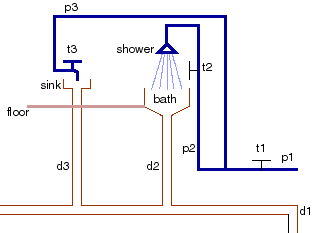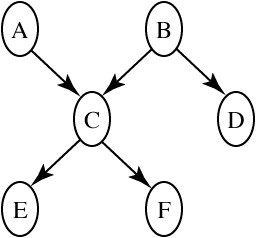CPSC 502 -- Fall 2006
Assignment 4
Due: 12:30pm, Monday 30 October 2006.

# Question 1

Consider the domain of house plumbing represented below. This is a simplified model of the same domain used in the previous assignment.

In this figure, p1, p2 and p3 denote cold water pipes. p1 is the pipe coming in from the main water supply. t1, t2 and t3 are taps and d1, d2 and d3 are drainage pipes. The constants shower denotes a shower, bath denotes a bath, sink denotes a sink, and floor denotes the floor. There can also be plugs in the sink or in the bath. You can assume that you are in a static situation (i.e., you don't have to worry about time); imagine that you have stumbled in this situation and must reason about it.

Suppose you can observe or query the tap positions, flow out of drain d1, and whether there is water in the sink and bath, and whether there is water on the floor.plumbing.gif

The Plumbing Domain

1. What are the random variables? In particular, you need random variables to represent the observations you may want to make, the queries you may be interested and other (hidden) variables to keep the model simple. For each variable, give its domain and intended interpretation.
2. Give a belief network for these variables, assuming a causal ordering of the variables. Give reasonable conditional probability tables. You can use the CIspace applet or any other belief network tool. (e.g., Netica or Genie).
3. Suppose you had to demonstrate to a skeptic that Belief networks are appropriate for this domain. Argue that this is a reasonable representation, and as part of your explanation, you need to give some test cases that show what your model is capable of. Use proper English. Be brief and concise (as this skeptic doesn't have much time).
4. What would your belief network look like if you had chosen the opposite ordering of variables?

# Question 2

Consider the belief network:abcdef.gif

with Boolean variables (we will write A=true as a and A=false as ¬a) and the following conditional probabilities:
 P(a) = 0.9 P(b) = 0.2 P(c|a,b) = 0.1 P(c|a,¬b) = 0.8 P(c|¬a,b) = 0.7 P(c|¬a,¬b) = 0.4 P(d| b) = 0.1 P(d|¬b) = 0.8 P(e|c) = 0.7 P(e|¬c) = 0.2 P(f|c) = 0.2 P(f|¬c) = 0.9
1. Compute P(e) using variable elimination. Please try to do it by hand, then check it with the CIspace applet. Do not do any pruning. Explain the significance of each factor created (either what does it represent or why does it have particular values).
2. Compute P(e|¬f) using variable elimination. How much of the previous computation can be reused? Show only what is different.
3. Compute P(a|d) using variable elimination.
4. Compute P(a | ¬f).
5. Compute P(a|¬f,d).
6. Explain when observing d affects your belief in a. (Be as specific as possible.)

# Question 3

Suppose you want to compute P(f|d) in the belief network of the previous question. You are to use this example to explain how each of the following work. (Assume you are writing a textbook for your peers, and are using this example to explain these concepts)
1. Rejection sampling
2. Importance sampling
3. Particle filtering

David Poole